Name:    2nd Grade Math Basic Test 4

Multiple Choice
Identify the choice that best completes the statement or answers the question.

1.

Measurement – Length, Height, Volume – RIT 151 – 160Which tree is tallest?
 a. 1 d. 4 b. 2 e. 5 c. 3

2.How long is this pencil?
 a. 7 cm d. 5 cm b. 6 cm e. 3 cm c. 4 cm

3.

Measurement – Time, Temperature – RIT 151 – 160
What would you use to tell time?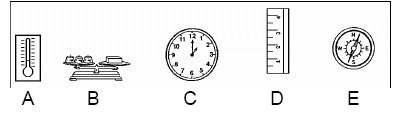a. A d. D b. B e. E c. C

4.

About what is the temperature on the thermometer?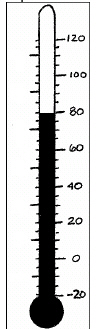a. 0° d. 80° b. 20° e. 50° c. 30°

5.

Measurement – Money – RIT 161 – 170How much is the coin worth?
 a. 5 d. 50 b. 10 e. 25 c. 1

6.

Measurement – Time, Temperature – RIT 161 – 170
What month comes next?
January, February,
 a. October d. March b. December e. April c. September

7.What time is it on the clock?
 a. 8:58 d. 10:15 b. 12:09 e. 5:23 c. 11:44

8.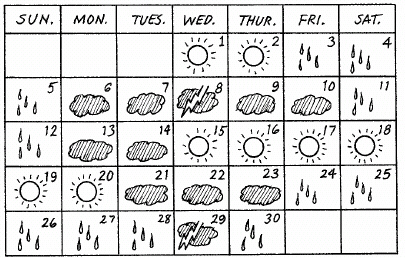What day of the week is the 1st?
 a. Friday d. Wednesday b. Sunday e. Saturday c. Tuesday

9.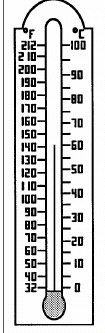What is the temperature in degrees Celsius?
 a. 800  C d. 600  C b. 1400  C e. 200  C c. 500  C

10.

Measurement – Area, Perimeter, Circumference – RIT 171 – 180About how many paper clips would it take to go around this shape?
 a. 7 d. 14 b. 10 e. 12 c. 4

11.

Find the area in square units.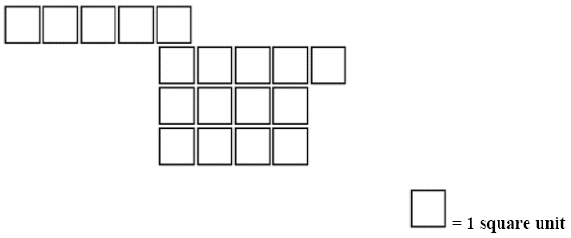a. 18 d. 12 b. 16 e. 24 c. 20

12.

Measurement – Length, Height, Volume – RIT 171 – 180
You can find these containers in your house.  Which one of these would hold the most water?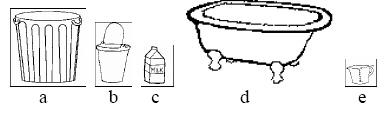a. e d. d b. c e. b c. a

13.

How long is the lightbulb?a. 4 inches d. 1 inch b. 3 inches e. 5 inches c. 2 inches

14.

How much do the potatoes weigh?a. 9 d. 0 b. 6 e. 4 c. 3

15.These 4 cups are the same as: ______
 a. 1 pint d. 2 quarts b. 1 gallon e. 3 pints c. 1 quart